# SUBSTITUTE Fx – Find / Replace Text – Excel, VBA & G Sheets

This Tutorial demonstrates how to use the Excel SUBSTITUTE Function in Excel to find and replace text.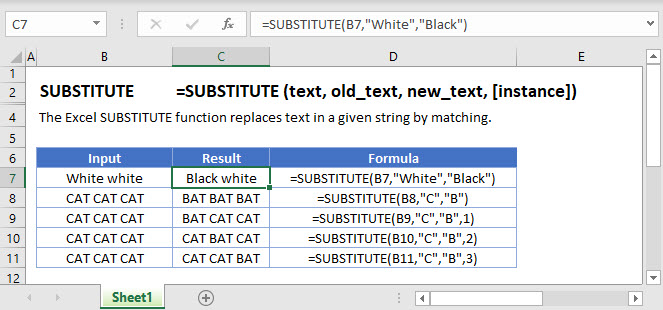## SUBSTITUTE Function Overview

The SUBSTITUTE Function Finds and replaces existing text with a new text string. Case-sensitive.

To use the SUBSTITUTE Excel Worksheet Function, select a cell and type: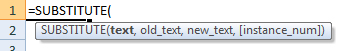(Notice how the formula inputs appear)

## SUBSTITUTE function Syntax and inputs:

``=SUBSTITUTE(text,old_text,new_text,instance_num)``

text – The original string of text.

old_text – The text string that you wish to find and replace.

new_test – The replacement text.

instance_num – OPTiONAL. The instance number to replace. If blank, all instances are replaced.

## What is SUBSTITUTE?

The SUBSTITUTE function in Excel is used to substitute a string of characters in a text string with a different specified text string.

Let us look at the following example substituting the string “quick brown” with “slow white”.

``=SUBSTITUTE(C2,C3,C4)``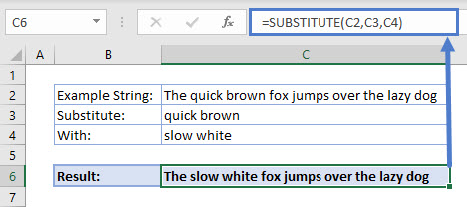SUBSTITUTE looks in the string in C2, finds “quick brown” and replaces it with “slow white”.

## How to use SUBSTITUTE

The SUBSTITUTE function takes 3 required arguments and 1 optional one:

• Text: The text that you want to replace characters
• Old_text: The text you want to replace
• New_text: The text you want to replace Old_text with
• Instance_num (optional): Specifies the occurrence of Old_text you want to replace with New_text. By default, all occurrences of Old_text will be replaced if an instance is not specified.

## Nesting SUBSTITUTE

What if we had to substitute two parts of the same string? Let us see how we would combine two SUBSTITUTE functions.

``=SUBSTITUTE(SUBSTITUTE(C2,C3,C4),C5,C6)``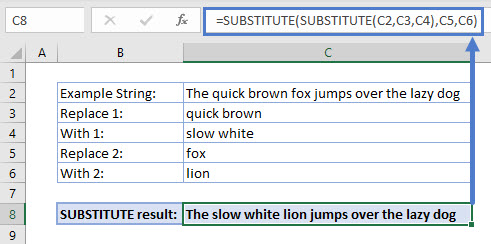Notice how the result of SUBSTITUTE(C2,C3,C4) is used as the first argument for the second SUBSTITUTE. If we break the formula apart:

=SUBSTITUTE(C2,C3,C4) evaluates to “The slow white fox jumps over the lazy dog”.

Then, SUBSTITUTE(“The slow white fox jumps over the lazy dog”, C5,C6) which evaluates to “The slow white lion jumps over the lazy dog”.

## Difference between SUBSTITUTE and REPLACE

A similar function to SUBSTITUTE is REPLACE. In most cases, these can be used interchangeably however, the following rule of thumb applies.

The SUBSTITUTE function should be used when the string of text to be replaced is known, or a whole word or words are being replaced, like our fox example used earlier.

``=SUBSTITUTE(C2,C3,C4)``The REPLACE function should be used when the position of the text characters to be replaced in the string are known, or when a part of a word or string is being replaced. For example, removing a hyphen from a string of numbers.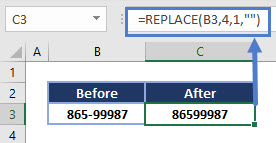The REPLACE function can also be nested like SUBSTITUTE however, it is important to note that the relative position of the start character may change based on the length of the string to be replaced in the first step of the nested formula.

For example, replacing “quick brown” with “slow white” is replacing 11 characters with 10 characters. Therefore, if nested, the starting character for the second REPLACE would be 1 character off from the initial character positions.

### Easy Excel Automation## SUBSTITUTE Tips

• SUBSTITUTE can be used on substrings: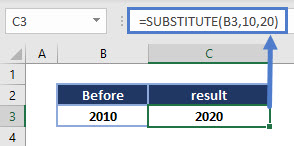• Remember you need to specify an instance_num if you are trying to substitute a set of characters that repeat in the string: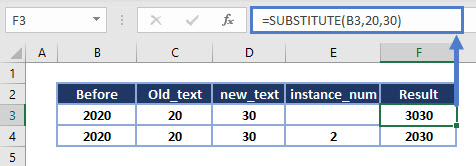Notice the 3030 is incorrect as both “20” and “20” in “2020” were replaced with “30”.

## Interested in More Text functions?

See our other articles for more on REPLACE, or how other text functions in Excel such as LEFT, MID and RIGHT are used.

The SUBSTITUTE Function works exactly the same in Google Sheets as in Excel: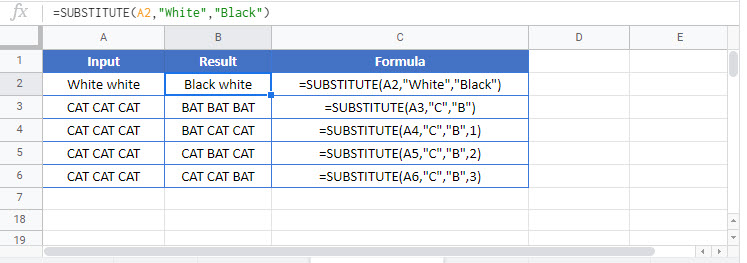The SUBSTITUTE Function is case sensitive!. You can use the LOWER or UPPER Functions to convert your strings of text into a consistant case before using SUBSTITUTE Function. Then, if desired, you can use the PROPER Function to capitalize the first letter of each word.

Alternatively, you can use the Functions SEARCH and REPLACE to simulate a non case-sensitive SUBSTITUTE. Use SEARCH Function to find the starting position of the text string. The SEARCH Function is not case-sensitive. Then use REPLACE Function to replace the text. You will need to use the result from the SEARCH Function as the start_num input in REPLACE. You will also need to define the number of characters to replace ( num_chars). You can manually count them or use the LEN Function to count the number of characters. Example:

### Substitute – Remove Hyphens

A common question is how to remove hyphens from text. The trick is to Substitute the hyphen with nothing.

For this example, let’s assume cell A1 has text with hyphens in it. Here’s how to remove them

1. In a blank helper cell type =SUBSTITUTE(
2. Click on the cell you would like to substitute characters in or A1 (this automatically fills your formula)
3. Type, (comma)
4. Type “-”
5. Type, (comma)
6. Type “” and hit enter
=SUBSTITUTE(A1,”-“,””)

### 3 More Substitute Examples:

Looking at cell B3, we want to replace the word “sick” with “large”. We can use the following expression:

``=SUBSTITUTE(B3,"sick","large",1)``

If the text that we want to replace occurs more than once then we need to specify the instance that we mean. In the second example, we have two occurences of the word “old”. So if we want to change second instance to the word “grey” then we have to use:

``=SUBSTITUTE(B4,"old","grey",2)``

Note that if the occurrence is not specified then all instances are replaced. So:

``=SUBSTITUTE(B4,"old","grey")``

Would get rid of all instances of the word “old” and replace them with the word “grey”. Note that if the old text cannot be found then the string is unchanged. So looking at the last example:

``=SUBSTITUTE(B5,"black","grey",1)``

Means that we try and replace the word “black” with the word “grey”. However, as “black” does not occur the original string remained levitra shipped in the united states unchanged:## SUBSTITUTE Examples in VBA

You can also use the SUBSTITUTE function in VBA. Type:

``application.worksheetfunction.substitute(text,old_text,new_text,instance_num)``

For the function arguments (text, etc.), you can either enter them directly into the function, or define variables to use instead.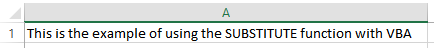When the following code is executed

``Range("A1") = WorksheetFunction.Substitute(Range("A1"), "using", "", 1)``

The contents of cell A1 will become: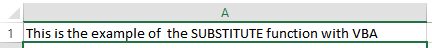So, the word “using” was replaced by an empty string. The last parameter (1) indicates which instance of the text should be replaced, in case the text is present more than one times. So, for example if we execute the following statement

``Range("A1") = WorksheetFunction.Substitute(Range("A1"), "the", "an", 1)``

The A1 cell will look like this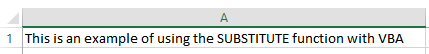Notice that the first occurrence of “the” was replaced by “an”, while the second “the” was not affected. If we omitted the last parameter, both “the” words would have been replaced